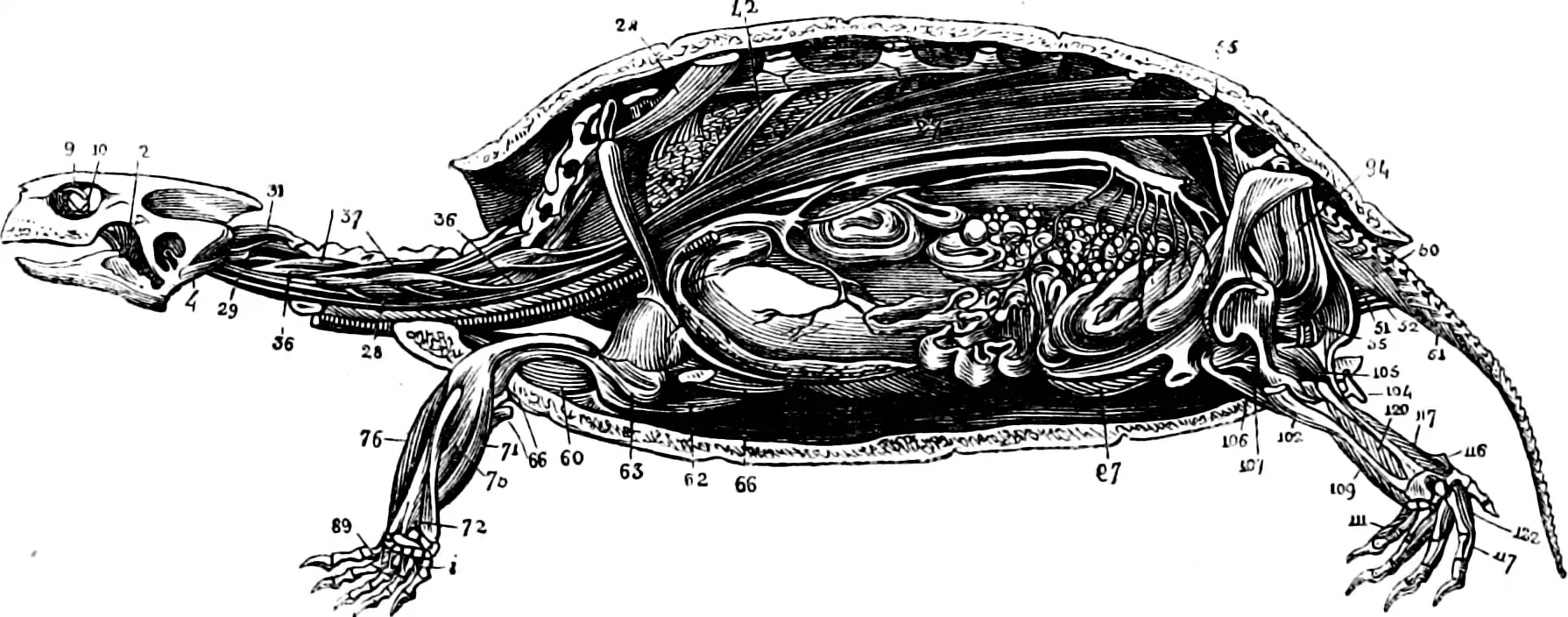# Bayesian inverse problemsInverse problem solution with a probabilistic approach. In Bayesian terms, say we have a model which gives us the density of a certain output observation $$y$$ for a given input $$x$$ which we write as $$p(y\mid x)$$. By Bayes’ rule we can find the density of inputs for a given observed output by $p(x \mid y)=\frac{p(x) p(y \mid x)}{p(y)}.$ The process of computing $p(x \mid y)$ is the most basic step of Bayesian inference, nothing special to see here.

In the world I live in, $$p(y \mid x)$$ is not completely specified, but is a regression density with unknown parameters $$\theta$$ that we must also learn, that may have prior densities of their own. Maybe I also wish to parameterise the density for the prior on $$x$$, $$p(x \mid \lambda),$$ which is typically independent of $$\theta.$$ Now the model is a hierarchical Bayes model, leading to a directed factorisation $p(x,y,\theta,\lambda)=p(\theta)p(\lambda)p(x\mid \lambda) p(y\mid x,\theta).$ We can use more Bayes rule to write the density of interest as $p(x, \theta, \lambda \mid y) \propto p(y \mid x, \theta)p(x \mid\lambda)p(\lambda)p(\theta).$ Solving this is also, I believe, sometimes called joint inversion. For my applications, we usually want to do this in two phases. In the first, we have some data set of $$N$$ input-output pairs indexed by $$i,$$ $$\mathcal{D}=\{(x_i, y_i:i=1,\dots,N)\}$$ which we use to estimate posterior density $$p(\theta,\lambda \mid \mathcal{D})$$ in some learning phase. Thereafter we only ever wish to find $$p(x, \theta, \lambda \mid y,\mathcal{D})$$ or possibly even $$p(x \mid y,\mathcal{D})$$ but either way do not thereafter update $$\theta, \lambda|\mathcal{D}$$.

If the problem is high dimensional, in the sense that $$x\in \mathbb{R}^n$$ for $$n$$ large and ill-posed, in the sense that, e.g. $$y\in\mathbb{R}^m$$ with $$n>m$$, we have a particular set of challenges which it is useful to group under the heading of functional inverse problems.1 A classic example of this class of problem is “What was the true image what was blurred to create this corrupted version?”.

## Laplace method

We can use Laplace approximation approximate latent density.

Laplace approximations seems like it might have an attractive feature: providing estimates also for inverse problems by leveraging the delta method. I think this should come out nice in network linearization approaches such as Foong et al. (2019) and Immer, Korzepa, and Bauer (2021).

Suppose we have a regression network that outputs (perhaps approximately) a Gaussian distribution for outputs given inputs.

TBC.1. There is also a strand of the literature which refers to any form of Bayesian inference as an inverse problem, but this usage does not draw a helpful distinction for me so I avoid it.↩︎

### No comments yet. Why not leave one?

GitHub-flavored Markdown & a sane subset of HTML is supported.